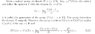# Commutation of Hamiltonian and time evolution operator

• bikashkanungo

#### bikashkanungo

Can anyone explain how the time evolution operator commutes with the Hamiltonian of a system ( given that the the Hamiltonian does not depend explicitly on t ) ?

Do you know how to express the time evolution operator in terms of H? (It's an exponential). Do you know the definition of an exponential?

yeah i know that U = exp[-iH*(t-t0)/ħ] if H does not depend explicitly on time .

Right. And the exponential is defined as a power series. So what is [H,U]? (You can choose t0=0 and units such that $\hbar=1$ to eliminate some typing).

Last edited:
The exponential is defined as a power series only if H is bounded. For H bounded (which is rarely the case in QM) the proof is trivial (purely algebraic). For the unbounded case, it should go like this. From the theory of Galilei group representation, one derives that time evolution of pure states is provided by means of a strongly continuous group of unitary operators U(t) and we agree to denote by H the generator of this group and call it Hamiltonian, which is time-independent, if one's working in the Schrödinger picture. To show that the generator commutes with U(t), we start from the definition of the generator (H) and I have attached a paragraph from Blank et al.

The fact that H as the generator is self-adjoint represents the conclusion of the theorem of Stone, which is found in most books on functional analysis.

#### Attachments

•Blank - excerpt.jpg
27 KB · Views: 1,663
Last edited:
Cool. I knew that Stone's theorem is what you use in the unbounded case, but I have never studied the details. I wasn't aware of the stuff mentioned in the attachment.

To a typical physics student, a rigorous treatment of the bounded case is not trivial. They aren't expected to understand (or even care about) how to prove that the series that defines the exponential is convergent given that H is bounded, or what sort of things they can do to series and other expressions that involve limits. A physics student who's given this problem is expected to answer it with $$[U(t),H]=\left[e^{-iHt},H\right]=\left[\sum_{k=0}^\infty\frac{(-iHt)^k}{k!},H\right]=\sum_{k=0}^\infty \frac{(-it)^k}{k!}[H^k,H]=0$$ without even thinking about whether these steps actually make sense.

Hmmm, a proof of strong convergence should be handy for a theoretical physics student, because he's normally taught (i.e. shown) in a class on real analysis that the Euler-MacLaurin series expansion of eax converges, once |a|=1 and x$\leq$1.

If he knows from functional analysis / math methods for quantum physics what a bounded operator is, he can make a proof of strong convergence, I believe.